# Abstract

In this paper, a general scheme for generating extra cuts during the execution of a Benders decomposition algorithm is presented. These cuts are based on feasible and infeasible master problem solutions generated by means of a heuristic. This article includes general guidelines and a case study with a fixed charge network design problem. Computational tests with instances of this problem show the efficiency of the strategy. The most important aspect of the proposed ideas is their generality, which allows them to be used in virtually any Benders decomposition implementation.

Benders decomposition; extra cuts generation; mixed-integer programming

Accelerating benders decomposition with heuristicmaster problem solutions

Alysson M. CostaI.* * Corresponding author ; Jean-François CordeauII; Bernard GendronIII; Gilbert LaporteII

IUniversidade de São Paulo, Av. do Trab. São Carlense, 400, CP 668, São Carlos, Brazil. E-mail: alysson@icmc.usp.b

IICIRRELT and HEC Montréal, Montréal H3T 2A7, Canada. E-mails: jean-francois.cordeau@cirrelt.ca / gilbert.laporte@cirrelt.ca

IIICIRRELT and Université de Montréal, Montréal H3C 3J7, Canada. E-mail: bernard.gendron@cirrelt.ca

ABSTRACT

In this paper, a general scheme for generating extra cuts during the execution of a Benders decomposition algorithm is presented. These cuts are based on feasible and infeasible master problem solutions generated by means of a heuristic. This article includes general guidelines and a case study with a fixed charge network design problem. Computational tests with instances of this problem show the efficiency of the strategy. The most important aspect of the proposed ideas is their generality, which allows them to be used in virtually any Benders decomposition implementation.

Keywords: Benders decomposition, extra cuts generation, mixed-integer programming.

1 INTRODUCTION

Benders decomposition (Benders, 1962) is a classical solution approach for combinatorial optimization problems based on the ideas of partition and delayed constraint generation. The method decomposes the model to be solved into two simpler formulations, called master problem and subproblem. The initial master problem is a relaxed version of the original problem, containingonly a subset of the variables and of the constraints. The subproblem is the original problem inwhich the variables considered in the master problem are fixed.

The Benders method relies on the iterative solution of the master problem and subproblem toobtain a provable optimum for the original model. The solution of the master problem gives atentative solution for the variables it contains and a dual bound. These variables are then fixedin the subproblem whose solution yields a valid cut for the master problem. The cut is calledan optimality cut if the partial solution found by the solution of the master problem is part of a complete feasible solution (in which case a primal bound is also obtained) or a feasibility cut ifthe fixing of the master problem partial solution yields an infeasible subproblem. This procedureis repeated until the gap between the dual and primal bounds is closed.

A large number of algorithmic improvements and modifications have been proposed to accelerate the convergence of the Benders decomposition method. Magnanti & Wong (1981) have studied the influence of optimality cuts in a Benders decomposition algorithm and have shown that the use of stronger cuts can have an important impact on the convergence of the algorithm byreducing the number of iterations. The main idea proposed by the authors is to make use of the existence of multiple optimal solutions to the dual of the subproblem to obtain better cuts, and isbased on the solution of an extra auxiliary problem with similar dimensions to the subproblem.Papadakos (2008) has enhanced this method to eliminate the necessity of solving the extra auxiliary problem. Rei et al. (2008), in turn, have used local branching to improve the quality ofthe bounds obtained through out the solution process. Other improvements have concentrated onspecific categories of problems. Wentges (1996), for instance, proposed a procedure for strengthening Benders' cuts in the context of the capacitated facility location problem. More recently, Costa et al. (2009) have shown some structural relationships between the Benders cuts obtained for general fixed-charge network design (FND) problems and some classical valid inequalities for that family of problems, such as cutset and metric inequalities. These relationships have enabled the strengthening of the obtained Benders cuts via the solution of simple shortest path problems.

Among the algorithmic enhancements that have been proposed for the Benders decomposition approach, the strategy of McDaniel & Devine (1977) is certainly one of the most popular. Theiridea consists in solving a relaxed version of the original problem in order to obtain a numberof valid cuts. Very often, the implementation of this idea is achieved by relaxing the integrality constraints on the master problem variables. The resolution of the master problem is usually themost time-consuming part of a Benders decomposition approach only because it is generally amixed-integer problem. Therefore, with the use of the linear programming (LP) relaxation, the authors are able to quickly find a large number of cuts during these so-called relaxed Bendersiterations. After the relaxed problem is solved, these cuts can be used in the process of solving the original problem, eliminating part of the search space and accelerating convergence. We callthe process of solving the relaxed Benders iterations relaxed Benders phase, in opposition to the subsequent integer Benders phase.

The improvements obtained with the strategy of McDaniel & Devine depend on the quality ofthe linear programming relaxation representation of the mixed-integer master problem. Indeed,it is not uncommon that after the LP problem is solved, only a few integer Benders iterations are needed before convergence. However, if the relaxed master problem yields solutions that do 3not have a strong correspondence with the integer master problem solutions, the efficiency of thestrategy may be severely reduced.

The purpose of the present article is to present procedures for accelerating the convergence of theBenders' decomposition algorithm. The main idea is to increase the number and the quality of the cuts to be added, especially during the relaxed Benders phase, in order to reduce the numberof iterations of the subsequent Benders integer phase. The implementation of this idea can beachieved in a number of ways (which are usually problem dependent), but the concept behind them is always the search for good tentative solutions that somehow mimic the solutions that are obtained in the integer Benders phase.

The remainder of this paper is organized as follows. In the next section we present a brief reviewof the Benders decomposition approach. Then, in Section 3, general guidelines for obtaining good tentative solutions are discussed. In Section 4, we present a brief case study, by special izingthe content discussed in the previous sections for a family of FND problems. Computational results showing the validity of the strategy are presented and discussed in Section 5. Section 6 closes this paper with general conclusions.

2 BENDERS DECOMPOSITION APPROACH

In this section, we present a brief explanation of the Benders decomposition method. For this,we use the general mixed-integer problem:The variables of the model are written in two vectors, x and y, which contain the continuous and the integer variables, respectively. Matrices A, B and D and vectors b and e have the appropriate dimensions. Problem (1)-(4) can be viewed as two nested minimization problems, one in each group of variables:where Y ={y|Dy e, y ≥ 0 and integer}. Observe that the inner minimization problem is alinear programming problem. Associating dual variables u to constraints Ax b B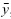, it is possible to write the dual of the inner minimization problem as: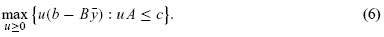Equation (6) is the Benders dual subproblem. Using duality theory, the primal and the dualformulations can be interchanged, and (5) can be rewritten as:This change is convenient because the feasible space of the inner maximization problem (thedual subproblem) then does not depend on the choice made for the y variables. Let F ={u|u ≥ 0 : uA c} represent this feasible space. We assume that F is not empty for it would correspond to an infeasible or to an unbounded primal problem. The set F is therefore composed of extreme points u Epand extreme rays r Er, where Epand Erare the sets of extreme points and extreme rays of F, respectively.

The solution of the dual subproblem can be either bounded or unbounded. In the first case, the solution is one of the extreme points u, otherwise, there is a direction r for which r(b B)> 0. The latter situation results in an infeasible primal problem and must be avoided (otherwise, the interchange between the primal and dual problems would not be valid). This is done by explicitly chosing y variables for which:With these aditional constraints, the solution of the inner problem is one of the extreme points of F. Problem (5) then becomes: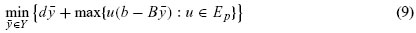or, with the use of a an extra continuous variable z:Model (11)-(14) is called the Benders reformulation and its main drawback is the fact that thenumber of extreme points and extreme rays of the subproblem is usually extremely large and ,therefore, the model potentially has a very large number of constraints. In order to overcome thisdifficulty, Benders proposed delaying the generation of constraints (12) and (13). Initially, onlythe constraints on the y variables (14) are considered, yielding the first master problem:As a relaxed version of the original problem, the objective value of a solution to model (15)-(16)gives a lower bound (LB) for the original formulation. The solution (on the y variables) is also atentative solution that can be used in the dual subproblem (6). This subproblem is solved and theresult is either unbounded, in which case a constraint of type (13) is found, or it is bounded andan extreme point leading to a constraint of type (12) is found. In the latter case, the conjunctionof the solution of the master with the primal solution of the subproblem provides a completesolution and, consequently, an upper bound (UB), for the original problem.

With the inclusion of the new generated constraints, of type (12) or (13), in the master problem,a new tentative solution on the y variables can be obtained and the process iterates until the obtained lower and upper bounds are sufficiently close (i.e., they differ by less than a predefined tolerance ε). Algorithm 1 presents a pseudo-code of the approach.

Algorithm 1

3 GENERAL GUIDELINES FOR OBTAINING GOOD TENTATIVE SOLUTIONS

The main idea of this paper is to quickly generate good tentative solutions that can be used toobtain good Benders cuts. This can be broadly expressed by the following two main guidelines:

• The tentative solutions should be generated with a reasonable computational effort.

• The tentative solutions should be similar to the solutions that would be obtained during theinteger Benders phase.

The first guideline is concerned with the fact that if it is too time-consuming to generate the tentative solutions, then the best method would probably be to run the integer master problem it self. The second guideline expresses the fact that the main goal of generating these tentative solutionsis to reduce the need for integer Benders iterations, which will happen if the cuts generated by the tentative solutions cut part of the search space that would otherwise only be eliminated with theuse of tentative solutions obtained by solving the mixed-integer version of the master problem.

We propose several general strategies that can be used to obtain cuts that respect these guidelines.We divide these strategies into two categories: strategies that can be used during the relaxedBenders phase, and strategies that are more appropriate for the integer Benders phase. These are described in the two following sections.

3.1 Strategies for the relaxed Benders algorithm phase

During the relaxed Benders phase, the solutions obtained by the master problem are most likely fractional and can, therefore, be poor approximations of the desired integer solutions. In thissection, we propose two general approaches that make use of these continuous solutions. Theaim is to obtain tentative integer solutions more likely to resemble the solutions that would be obtained during the integer Benders phase. The main motivation for these methods is presentedin the following. We also discuss some implementation concerns arising when specializing the semethods for particular problems.

3.1.1 Rounding off strategies

The literature is rich in methods that use fractional solutions obtained by the simplex method(or any other linear programming method) to obtain approximations of integer solutions. These approaches are usually called rounding-off or LP-rounding methods and have been successfully used in a wide range of different combinatorial problems (Bansal et al., 2010; Byrka, Srinivasan & Swamy, 2010; Thanh, Bostel & Péton, 2011). The idea of these methods is to round offractional solutions in order to obtain feasible integer solutions. This LP-rounding strategy can make use of the problem particularities or of any existing knowledge about the structure of good solutions.

The adaptation of this strategy to the context of generating better Benders cuts is straight forward.The rounded solution (obtained with any possible rounding-off strategy) can be directly used in the subproblem, whose solution will yield a new Benders cut.

Implementation issues: One interesting aspect of any implementation of rounding-off strategies to generate extra cuts is the fact that it is not necessary to guarantee that the new solution completely respects the integrality constraints. Indeed, the feasibility of the master problem solution with respect to any constraint is not required to ensure the generation of valid cuts. As a result, the method may work with integral (but otherwise not feasible) solutions, or feasible solutions with respect to the other constraints (but not integrality) in order to find a wide range of tentative solutions. In other words, partial rounded solutions (with some fractional values) or complete rounded solutions can be used. More over, these (partially) rounded solutions can either respect or not respect the master problem constraints. In all (four) cases, the set of master variables can be used in the subproblem to generate a cut. Along these lines, a simple strategy is to round off the variables to their closest integer, one at a time, to generate tentative solutions. For computational efficiency reasons and in order to limit the number of tentative solutions generated, one can use a parameter indicating the maximum number of solutions for each fractional solution ora thre shold used to decide which variables should be rounded.

3.1.2 Cost-scaling strategies

Dynamic cost-scaling (or slope-scaling) algorithms have been used with success to approximately solve combinatorial optimization problems (Gendron, Potvin & Soriano, 2003; Kim &Pardalos, 1999). The main idea of the method is to iteratively solve relaxed versions of a mixed-integer problem containing only the continuous variables. At each new iteration, the objective function is updated to reflect the real cost that would be incurred if the cost of the integer variables was considered. The method is known as slope-scaling because the obtention of the new costs at each iteration corresponds to finding a slope that appropriately reflects the costs for both continuous and integer variables in a linear fashion.

The application of slope-scaling strategies to find better tentative solutions in the context of a Benders decomposition approach is also straight forward. If a slope-scaling heuristic is availablefor the problem, it can be initialized with the current fractional solution, and any solution found can be used as a tentative solution in the subproblem.

Implementation issues: As for the LP-rounding strategies, neither integrality nor feasibility with respect to the other constraints is needed to use a master problem solution for the purpose of obtaining a cut from the subproblem. Therefore, any intermediate slope-scaling solution found can be used as a tentative solution. The method must control the number of solutions used. This can be done, for example, by setting a threshold on the quality of the solutions before they canbe used as tentative solutions.

3.2 Strategies for the integer Benders algorithm phase

Extra cuts can also be obtained during the integer Benders phase. In this case, it is more likely that the extra tentative solutions are of good quality. We propose two strategies that rely on general concepts and can therefore be applied to a wide variety of situations.

3.2.1 Intermediate branch-and-cut solutions

The branch-and-cut method used in mixed-integer linear programming solvers has the feature of generating intermediate feasible solutions, which are used to reduce the search tree. The final result obtained by the method is a provable optimum. Nevertheless, the intermediate solutions are also easily accessible by the user in most implementations of the algorithm.

In the context of a Benders decomposition algorithm, these intermediate solutions obtained during the solution of the mixed-integer master problem can be used in the subproblem to generate feasibility or optimality cuts.

Implementation issues: Since there can be a large number of intermediate solutions, it may be interesting to only call to the subproblem at the last solutions obtained during the search, or at the solutions respecting a certain quality threshold. One important aspect is that the intermediate solutions can even be part of optimal solutions to the original problem (since, at this point, are laxed version of the master problem is being solved) and therefore these solutions usually provide good quality cuts.

3.2.2 Local search solutions

Once a solution is found, any neighbourhood-based strategy can be used to obtain similar but different solutions. As before, these new solutions can be used to generate new cuts to the problem. Note that because we have a relaxed master problem, neighbor solutions at this point may, in fact, be the optimal solution of the original problem. Moreover, neighbor solutions (even of lower quality) might help to more thoroughly cut the solution space at a given point of the iterative Benders procedure.

A number of methods can be applied to generate such extra solutions from an initial integer solution found by the branch-and-cut algorithm, such as simulated annealing (Kirkpatrick, 1984), tabu search (Glover, 1989a; 1989b) and variable neighborhood search (Mladenovic & Hansen,1997).

Implementation issues: The search for new neighbouring solutions can be done after the branch-and-cut algorithm converges, or iteratively during the enumeration process. The iterative approach can make use of extra processor cores (which are now commonly found even in low-costmachines). One interesting aspect is that if a better quality solution is found by the heuristic method, it can be used within the branch-and-cut process to accelerate its own convergence.

A final note concerns the management of the added cuts. In order to reduce the computational burden associated with solving the master problem, cuts added earlier in the procedure or cuts that have not been active for a large number of iterations might be removed from the problem. Nevertheless, in our computational experiments we observed that it usually paid off to keep all cuts in the problem, for the gain associated with saving just one Benders integer iteration usually compensates for the additional time spent solving the master problem.

4 A CASE STUDY WITH A FAMILY OF NETWORK DESIGN PROBLEMS

We now present a specialization of the ideas described in the last section to general FND problems. The choice of this family of problems is motivated by its importance (both theoretical and practical) in the field of operations research and by the fact that many Benders decomposition approaches have been designed with success to solve a large number of variants of these problems (Costa, 2005).

4.1 Problem definition and formulation

Let G = (V, E) be a graph where V is the set of vertices and E is the set of edges. Let also K be the set of commodities, each commodity k K being represented by a triplet (O(k), D(k), d(k)), where O(k), D(k) and d(k) are the origin vertex, the destination vertex andthe commodity demand, respectively. With each edge (i, j) ∈ E is associated a capacity wij ≥ 0,and a set of costs, representing the fixed cost of constructing (selecting) the edge, fij≥ 0, and the variable costs of transporting one unity of commodity k through the edge, cikj ≥ 0. Withthis notation, the general FND problem can be described as the problem of selecting edges in E in order to enable the flow of commodities k K from the origin to the destination, while respecting the edge capacities and minimizing the total network cost. Let xikj be a continuous variable designating the flow of commodity k on edge (i, j) and binary variables yijequal to one if egde (i, j) is selected, and to zero otherwise. A mixed-integer formulation for this problem is as follows: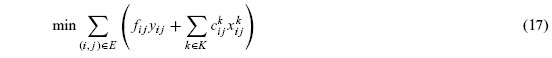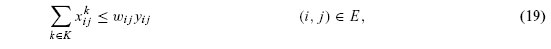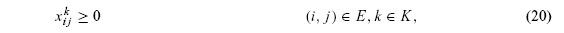This formulation suggests a natural Benders partitioning scheme which keeps the edge selection variables in the master problem and relegates the continuous variables to the subproblem. In this case, a natural interpretation of the Benders approach is possible: the master problem selects the edges in the network that should be constructed and the subproblem evaluates this solution in terms of its capacity and cost of transporting the demands.

Following the developments presented in Section 2, the subproblem can be written as: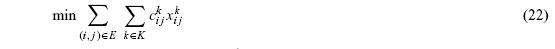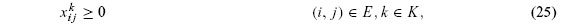and associating dual variables π and α to constraints (23) and (24), the master problem can be written as: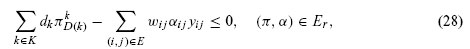where Erand Epare the sets of extreme rays and extreme points associated with the dual feasible space of the subproblem (22)-(25).

A standard Benders approach can be easily implemented with these developments and the general scheme presented in Algorithm 1, with the specialized versions of the cuts  equations (27) and (28)  being used in place of equations (12) and (13). In the following subsection we describe how the generation of extra cuts (line 7 in the pseudo-code) can be done in this particular case,as a specialization of the general guidelines presented in Section 3.

4.2 Generating extra cuts

In the following two sections, we describe the implemented strategies for generating extra cuts during the relaxed Benders phase (Subsection 4.2.1) and during the integer Benders phase (Subsection 4.2.2). Finally, in Subsection 4.2.3, a general view of the complete algorithm is presented.

4.2.1 Generating extra cuts during the relaxed Benders phase

We first describe how extra cuts can be generated during the relaxed Benders phase. At this stage, each master iteration gives a tentative fractional y solution. As described in Section 3, an easy strategy to approximate this tentative solution to an integer one is by rounding the fractional y variables. Algorithm 2 (which should be called in line 7 of the relaxed phase of Algorithm 1) presents a simple rounding strategy which gives priority to fractional variables with high values during the rounding process.

The stopping criterion can be based on the number of cuts already generated or on the values of the fractional variables (for example, generating one cut for each fractional variable greater than a given threshold). If a complete feasible solution is found, a call can be made to a slope-scaling algorithm (line 10). This algorithm can be used to generate further extra cuts and its pseudo-code is presented in Algorithm 3.

The modified version of problem (17)-(21) solved in lines 3 and 17 is the basis of the slope-scaling approach. It sets all y variables at one and modifies the objective function such that its value with the current x solution (ikj ) is equal to the original cost of (17) (Crainic, Gendron & Hernu, 2004). The objective function (17) then becomes:where mki j is the correction factor used to obtain the current total cost (including the fixed costs) and is given by: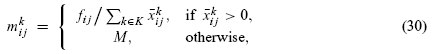where M is a large number. The problem is solved repeatedly until the new obtained solution does not change parameters mikj . In order to enlarge the set of solutions searched, Crainic, Gendron & Hernu (2004) suggest using the cost of the previous iteration instead of M, if there is no flow of commodity k in edge (i, j) in the current iteration.

The algorithm solves the problem with the slope scaling algorithm for the current solution (lines 2-4) but also solves c different problems where the values of variables yijassociated with edges (i, j) with large relative costs (given by the ratio between its fixed cost and its current flow line 13) are set to zero, one at a time (lines 12-27). The idea, again, is to follow the guideline that suggests that one should look for solutions which try to mimic solutions obtained in the integer Benders phase. Indeed, edges with a high ratio between fixed cost and flow tend to be less frequent in integer solutions.

4.2.2 Generating extra cuts during the integer Benders phase

The generation of extra cuts with feasible solutions found during the implicit enumeration method used to solve the master problem is easily implemented with simple modifications in Algorithm 1. Algorithm 4 shows the new pseudo-code.

As presented above, instead of waiting for the obtention of the master problem optimal solution,each new feasible solution found is used to generate cuts (line 3). However, these cuts are only added to the master problem once the optimal solution has been found (line 14). These cuts could be added iteratively, but adding the cuts at the end presented two main advantages: i)since the subproblem, in this case, is only solved after the master problem ends its execution, the computer implementation of the algorithm is much easier and does not require multiple solver licenses or special memory requirements, ii) adding the cuts iteratively during the execution of the algorithm could cause the premature convergence of the branch-and-bound, which could prevent finding other interesting master problem tentative solutions.

Whenever the tentative solution yields a feasible subproblem, Algorithm 3 can also be used to generate new cuts.

4.2.3 General view of the complete algorithm

The complete algorithm can be simply described as follows. During the relaxed Benders phase,Algorithm 1 is executed and, in line 7, a call is made to Algorithm 2 if the current subproblem is infeasible or to Algorithm 3 otherwise. Once the optimality gap is within a certain tolerance, the integer Benders phase starts with a call to Algorithm 4. Extra cuts are generated with the use of feasible (but not optimal) master problem solutions and, as before, line 7 calls Algorithm 3 whenever a feasible subproblem is solved.

5 COMPUTATIONAL RESULTS

We have tested the implementation described in the previous section with instances for the FND problem. Our computational experiments are based on a set of instances widely used in the FND literature, and described in detail by Ghamlouche, Crainic & Gendreau (2004). The algorithms were coded in C++ and the linear problems are solved with the use of the commercial package CPLEX 9.1. We have used a Pentium 4 computer with a 3GHz CPU and 512 kb of memory.

For each instance, a maximum computation time of two hours was allowed. Preliminary tests showed that when generating extra cuts via Algorithms 2 and 3, the first extra cuts were the most effective; therefore, the stopping criterion used was the number of cuts added. This number was equal to the minimum between 5 and the number of fractional y variables in the initial relaxed master problem solution.

In the table below, we present the results obtained by the Benders algorithm with and without the use of extra cuts. The goal of the study was not to obtain the most effective way to solve these instances (in which case several other Benders features could have been added) but rather to evaluate the performance improvement caused by the proposed strategy of extra cuts generation.

In the table, for each situation (with or without extra cuts), we present the computational timeneeded to solve the instance (time) and the final solution gap. Since the strategy proposed by McDaniel & Devine (1977) is used in both cases, we also list the number of Benders relaxed andinteger iterations (columns LP and IP, respectively) in each case.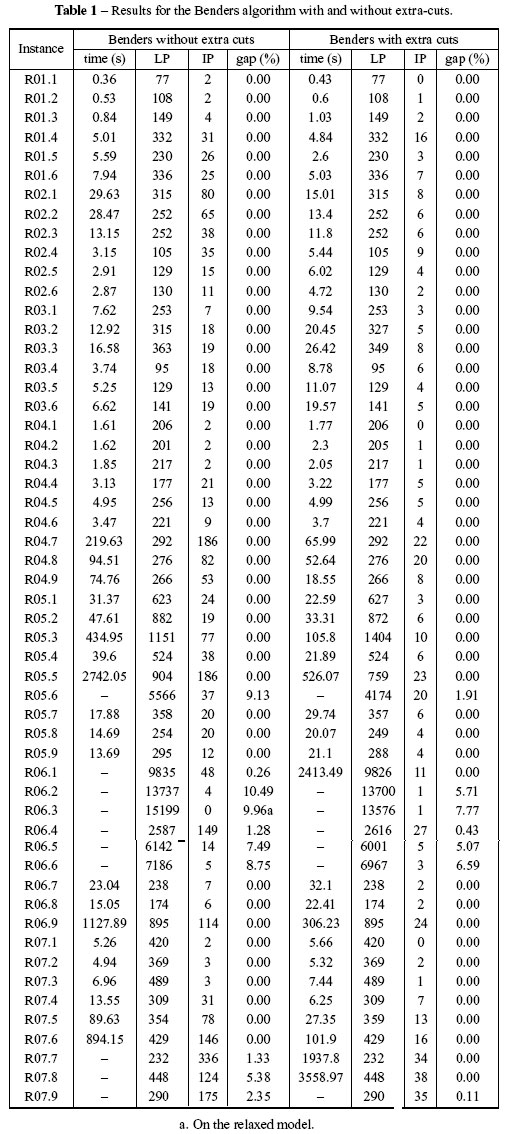A total of 54 instances were used. The number of iterations in the two versions of the method shows the efficacy of the extra cuts generation in eliminating the need for integer iterations. When both methods found an optimal solution, the average number of IP iterations dropped from 36 (when no extra cuts were used) to 6.6. One interesting aspect is the fact that the number of LP iterations remains very stable, indicating that the extra cuts are, indeed, working in a different area of the feasible space.

The reduction in the number of IP iterations had a positive effect on the computational times. Again, for the instances that were solved by both versions, the average computational time dropped from 138s in the case without extra cuts to 38s in the case with extra cuts. This effect was even more visible for the harder instances. The method without extra cuts needed morethan 1000s on average to solve instances R04.7, R05.3, R05.5, R06.9 and R07.6 while only 221s were needed to solve the same instances when extra cuts were generated.

For 10 instances, the method without extra cuts was not able to prove optimality in the allowed time of two hours. For three of these instances, the generation of extra cuts allowed the obtention of provable optima (on an average computational time of 2636s). For the remaining instances, the generation of extra cuts was able to halve the gaps (from 6.6% to 3.3%, on average). We exclude from this analysis instance R06.3 for which the original method was not even able to solve the relaxed version of the problem, while the generation of extra cuts allowed the obtention of an integer solution with a 7.8% gap.

Finally, in what concerns the efficacy of each procedure in improving the algorithm, it was clear that each family of extra cuts had its own importance in the reduction of costly integer iterations. Indeed, although the quality of cuts such as those obtained via slope scaling tended to be higher, the sole fact of cutting the space (even with less elaborate cuts) was enough to improve convergence and no definitive conclusions could be drawn on the dominance of one family of cuts over the other.

6 CONCLUSIONS

We have proposed a general scheme for generating extra cuts in a Benders decomposition approach, including general guidelines and a case study with an important family of problems. The most important aspect of the proposed ideas is their generality, which allows them to be used in virtually any Benders decomposition implementation. Computational tests on fixed charge network design instances suggest that the strategy is beneficial, improving the efficiency of a general Benders decomposition implementation.

ACKNOWLEDGMENTS

This research was supported by CAPES, CNPq and FAPESP. This support is gratefully acknowledged. We also thank two anonymous referees for their valuable suggestions.

Received April 6, 2011 / Accepted November 4, 2011

• 1. BANSAL N, GUPTA A, LI J, MESTRE J, NAGARAJAN V & RUDRA A. 2010. When LP is the cure for your matching woes: improved bounds for stochastic matchings. In: BERG M & MEYER U (Eds.), Lecture Notes in Computer Science (Vol. 6347, pp. 218-229). Berlin, Heidelberg: Springer, Berlin and Heidelberg.
• 2. BENDERS JF. 1962. Partitioning procedures for solving mixed-variables programming problems.Numerische Mathematik, 4: 238252.
• 3. BYRKA J, SRINIVASAN A & SWAMY C. 2010. Fault-tolerant facility location: a randomized dependent LP-rounding algorithm. In: F. Eisenbrand & F.B. Shepherd (Eds.), Lecture Notes in Computer Science (Vol. 6080, pp. 244-257). Berlin, Heidelberg: Springer, Berlin and Heidelberg.
• 4. COSTA AM. 2005. A survey on benders decomposition applied to fixed-charge network design problems. Computers & Operations Research, 32: 14291450.
• 5. COSTA AM, CORDEAU J-F & GENDRON B. 2009. Benders, metric and cutset inequalities for multi-commodity capacitated network design. Computational Optimization and Applications, 42: 371392.
• 6. CRAINIC TG, GENDRON B & HERNU G. 2004. A slope scaling/Lagrangean perturbation heuristic with long-term memory for multicommodity capacitated fixed-charge network design. Journal of Heuristics, 10: 525545.
• 7. GENDRON B, POTVIN J-Y & SORIANO P. 2003. A tabu search with slope scaling for the multicommodity capacitated location problem with balancing requirements. Annals of Operations Research, 122: 193217.
• 8. GHAMLOUCHE I, CRAINIC TG & GENDREAU M. 2004. Path relinking, cycle-based neighbourhoods and capacitated multicommodity network design. Annals of Operations Research, 131: 109133.
• 9. GLOVER F. 1989a. Tabu search Part I. ORSA Journal on Computing, 1: 190206.
• 10. GLOVER F. 1989b. Tabu search Part II. ORSA Journal on Computing, 2: 432.
• 11. KIM D & PARDALOS PM. 1999. A solution approach to the fixed charge network flow problem using a dynamic slope scaling procedure. Operations Research Letters, 24: 195203.
• 12. KIRKPATRICK S. 1984. Optimization by simulated annealing: quantitative studies. Journal of Statistical Physics, 34: 975986.
• 13. MAGNANTI T & WONG R. 1981. Accelerating Benders decomposition: algorithmic enhacement and model selection criteria. Operations Research, 23: 464484.
• 14. MCDANIEL D & DEVINE M. 1977. A modified Benders' partitioning algorithm for mixed integer programming. Management Science, 24: 312319.
• 15. MLADENOVIC N & HANSEN P. 1997. Variable neighborhood search. Computers & Operations Research, 24: 10971100.
• 16. PAPADAKOS N. 2008. Practical enhancements to the Magnanti-Wong method. Operations Research Letters, 36: 444449.
• 17. REI W, CORDEAU J-F, GENDREAU M & SORIANO P. 2008. Accelerating Benders decomposition by local branching. INFORMS Journal on Computing, 21: 333345.
• 18. THANH PN, BOSTEL N & PÉTON O. 2012. A DC programming heuristic applied to the logistics network design problem. International Journal of Production Economics, 135: 94105.
• 19. WENTGES P. 1996. Accelerating Benders' decomposition for the capacitated facility location problem. Mathematical Models of Operations Research, 44: 267290.
• *
Corresponding author

# Publication Dates

• Publication in this collection
08 Mar 2012
• Date of issue
Apr 2012